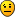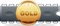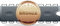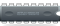## PID controller

Here you can post / find ECU expressions.

### PID controller

Thought i would post this to get some good explanations of what PID controller means, what it does, how do you change it, etc. My understand is only a little about it, and cant find enough info about it on the internet.VAG'dPosts: 102
Joined: Sun May 29, 2016 11:46 am

### Re: PID controller

Firstly, I apologize to everyone for mixing trivial terms with technical expression and sounding overall lame. It's midnight here.

In the field of closed loop process control technology, the PID controller is one of the first "complete" control systems, which allows for exact and speedy regulation of a process according to a set parameter.

PID controller thus takes an input DESIRED value (sollwert, "I'd-like-this-value-please", an input FEEDBACK value (istwert in Deutsch, "the-real-value-at-the-moment"), and makes an output value to control the process.

Usually, an error value is created by subtracting the desired from an actual value. Or you can have a different configuration and multiple inputs influencing the controller in different ways. Let's say we have a PID regulator (controller, depending on where you live), configured to control (regulate) temperature.

You set the value to, say 800°C. The input feedback value is 25°C. Thus the error difference is 775°C. Let's pretend that our PID controller (regulator) has an output regulating power from 0 to 1000W.

Now, the PID control equation (P.S. you can make a PID control loop in analog as well, usually with an operational amplifier, and the resulting circuit is just some sort of an amplifier, and you should see it to understand!!!):
y - output, x - error value input, t - time

y = P*x + I*x*t + D*dx/dt
(errors in here, sorry)

The proportional term of the control equation, P is "how much output should I put out in advance, directly in proportion of the difference of where I am and where I want to be."
The Integral or Integrational constant is, "how much should I be increasing the output per unit of error per time, thus, at a constant error, the contribution to the output value will be constantly rising, with rate of rise determined by the "I" constant.
And the Derivative or Differential constant is the most dangerous, because, while it can help, too much of it and your control system will start to oscillate. Why? Because the faster the error rate changes (yes, it reacts only to the speed by which your error value changes, it is zero is the error stays the same), the higher will the contribution to the output be.

lyeconPosts: 45
Joined: Mon Oct 02, 2017 11:00 pm
Location: garage pit

### Re: PID controller

Thank you for the explanation, as a newbie here it helped understand PID.

NoFacePosts: 3
Joined: Sat Nov 04, 2017 9:21 pm
Location: Portugal